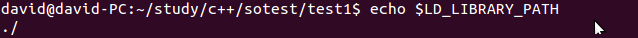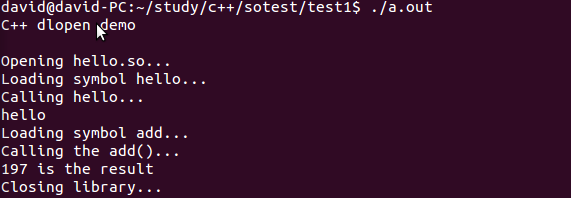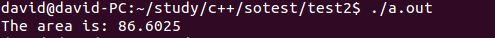# C++调用自己的.so

1. dlopen加载c++ 函数及类： http://blog.csdn.net/lwj1396/article/details/5204484

3. 动态调用动态库方法 .so: http://blog.csdn.net/lbmygf/article/details/7401862

### 然后从介绍Name Mangling开始

C++有个特定的关键字用来声明采用C binding的函数：extern “C” 。 用 extern “C”声明的函数将使用函数名作符号名，就像C函数一样。因此，只有非成员函数才能被声明为extern “C”，并且不能被重载。尽管限制多多，extern “C”函数还是非常有用，因为它们可以象C函数一样被dlopen动态加载。冠以extern “C”限定符后，并不意味着函数中无法使用C++代码了，相反，它仍然是一个完全的C++函数，可以使用任何C++特性和各种类型的参数。

### 示例程序1. 加载简单函数main.cpp的代码如下

[cpp]
//———-
//main.cpp:
//———-
#include
#include

int main() {
using std::cout;
using std::cerr;

cout << "C++ dlopen demo\n\n";

// open the library
cout << "Opening hello.so…\n";
void* handle = dlopen("libhello.so", RTLD_LAZY);

if (!handle) {
cerr << "Cannot open library: " << dlerror() << ‘\n’;
return 1;
}

typedef void (*hello_t)();

// reset errors
dlerror();
hello_t hello = (hello_t) dlsym(handle, "hello");
const char *dlsym_error = dlerror();
if (dlsym_error) {
cerr << "Cannot load symbol ‘hello’: " << dlsym_error <<
‘\n’;
dlclose(handle);
return 1;
}

// use it to do the calculation
cout << "Calling hello…\n";
hello();

// reset errors
dlerror();
if (dlsym_error) {
‘\n’;
dlclose(handle);
return 1;
}

// use it to do the calculation
cout << add(98, 99) << " is the result\n";

// close the library
cout << "Closing library…\n";
dlclose(handle);
}

[/cpp]

[cpp]
//———-
// hello.cpp:
//———-
#include

extern "C" void hello() {
std::cout << "hello" << ‘\n’;
}

extern "C" int add(int a, int b){
return a + b;
}
[/cpp]

1)       dlopen

a.根据环境变量LD_LIBRARY_PATH查找

b.根据/etc/ld.so.cache查找

c.查找依次在/lib和/usr/lib目录查找。

flag参数表示处理未定义函数的方式，可以使用RTLD_LAZY或RTLD_NOW。RTLD_LAZY表示暂时不去处理未定义函数，先把库装载到内存，等用到没定义的函数再说；RTLD_NOW表示马上检查是否存在未定义的函数，若存在，则dlopen以失败告终。

2)       dlerror

3)       dlsym

4)       dlclose

\$ g++ -shared -fPIC -o libhello.so hello.cpp
\$ g++ main.cpp -ldl### 示例程序2. 加载类

———————————-polygon.hpp—————————————————————

[cpp]
//———-
//polygon.hpp:
//———-
#ifndef POLYGON_HPP
#define POLYGON_HPP

class polygon {
protected:
double side_length_;

public:
polygon()
: side_length_(0) {}

virtual ~polygon() {}

void set_side_length(double side_length) {
side_length_ = side_length;
}

virtual double area() const = 0;
};

// the types of the class factories
typedef polygon* create_t();
typedef void destroy_t(polygon*);

#endif

[/cpp]

=0表示这个成员函数是纯虚函数，也就是它可以没有定义，只有接口，由它的继承类具体定义它的行为，当然，你也可以给它定义缺省的函数体

create_t* create_triangle = (create_t*) dlsym(triangle, “create”);

[cpp]
[/cpp]

———————————————-main.cpp———————————————-

[cpp]
//———-
//main.cpp:
//———-
#include "polygon.hpp"
#include
#include

int main() {
using std::cout;
using std::cerr;

void* triangle = dlopen("triangle.so", RTLD_LAZY);
if (!triangle) {
cerr << "Cannot load library: " << dlerror() << ‘\n’;
return 1;
}

// reset errors
dlerror();

create_t* create_triangle = (create_t*) dlsym(triangle, "create");
const char* dlsym_error = dlerror();
if (dlsym_error) {
cerr << "Cannot load symbol create: " << dlsym_error << ‘\n’;
return 1;
}

destroy_t* destroy_triangle = (destroy_t*) dlsym(triangle, "destroy");
dlsym_error = dlerror();
if (dlsym_error) {
cerr << "Cannot load symbol destroy: " << dlsym_error << ‘\n’;
return 1;
}

// create an instance of the class
polygon* poly = create_triangle();

// use the class
poly->set_side_length(10);
cout << "The area is: " << poly->area() << ‘\n’;

// destroy the class
destroy_triangle(poly);

dlclose(triangle);
}
[/cpp]

———————————————-triangle.cpp———————————————-

[cpp]
//———-
//triangle.cpp:
//———-
#include "polygon.hpp"
#include

class triangle : public polygon {
public:
virtual double area() const {
return side_length_ * side_length_ * sqrt(3) / 2;
}
};

// the class factories
extern "C" polygon* create() {
return new triangle;
}

extern "C" void destroy(polygon* p) {
delete p;
}

[/cpp]

go on..

\$ g++ -shared -fPIC -o triangle.so triangle.cpp
\$ g++ main.cpp -ldl
\$ ./a.out## 3 thoughts on “C++调用自己的.so”

1.google authorship code

When some one searches for his necessary thing, therefore
he/she wants to be available that in detail, therefore that thing
is maintained over here.

2.Anonymous

mm

1.David Post author

WTF

This site uses Akismet to reduce spam. Learn how your comment data is processed.